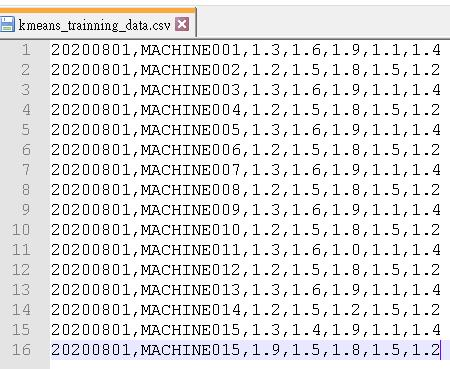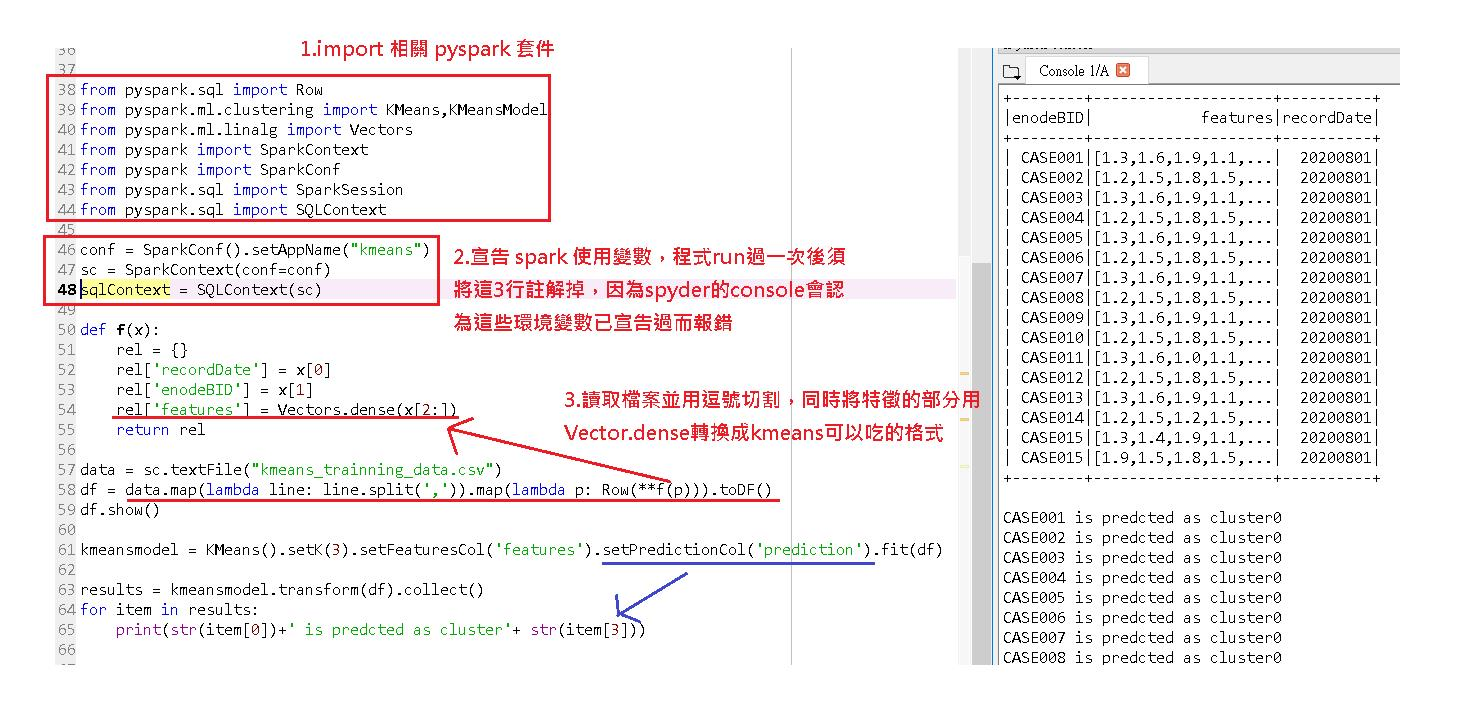### 利用 python 撰寫 KMeans 做分群

Coding KMeans Clustering Using Python with Spark。

## 1.資料集## 2.程式碼圖解說明ValueError: Cannot run multiple SparkContexts at once; existing SparkContext(app=kmeans, master=local[*]) created by __init__

## 3.程式碼

from pyspark.sql import Row
from pyspark.ml.clustering import KMeans,KMeansModel
from pyspark.ml.linalg import Vectors
from pyspark import SparkContext
from pyspark import SparkConf
from pyspark.sql import SparkSession
from pyspark.sql import SQLContext

conf = SparkConf().setAppName("kmeans")
sc = SparkContext(conf=conf)
sqlContext = SQLContext(sc)

def f(x):
rel = {}
rel['recordDate'] = x
rel['enodeBID'] = x
rel['features'] = Vectors.dense(x[2:])
return rel

data = sc.textFile("kmeans_trainning_data.csv")
df = data.map(lambda line: line.split(',')).map(lambda p: Row(**f(p))).toDF()
df.show()

kmeansmodel = KMeans().setK(3).setFeaturesCol('features').setPredictionCol('prediction').fit(df)

results = kmeansmodel.transform(df).collect()
for item in results:
print(str(item)+' is predcted as cluster'+ str(item))